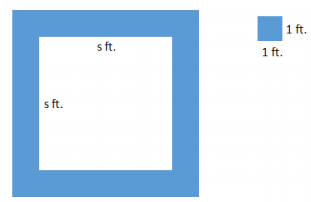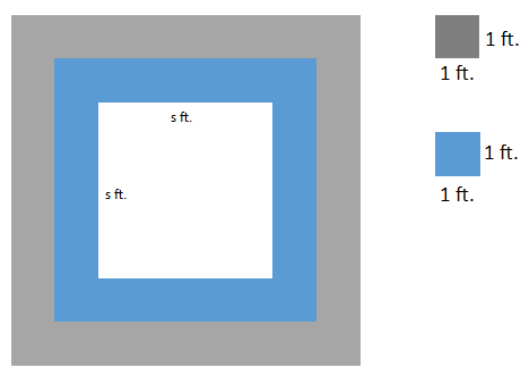# Numerical and Algebraic Expressions

## Objective

Write and interpret expressions in different ways to shed new meaning on a context.

## Common Core Standards

### Core Standards

?

• 7.EE.A.2 — Understand that rewriting an expression in different forms in a problem context can shed light on the problem and how the quantities in it are related. For example, a + 0.05a = 1.05a means that "increase by 5%" is the same as "multiply by 1.05."

?

• 6.EE.A.3

## Criteria for Success

?

1. Write multiple equivalent expressions to represent a situation.
2. Interpret each expression as it relates to the situation.
3. Understand how different expressions are interpreted differently in a situation.

## Tips for Teachers

?

This lesson touches upon standard 7.EE.2; this standard will be revisited in Unit 5 with percentages and other related contexts.

#### Fishtank Plus

• Problem Set
• Student Handout Editor
• Vocabulary Package

## Anchor Problems

?

### Problem 1

Malia is at an amusement park. She bought 14 tickets, and each ride requires 2 tickets.

1. Write an expression that gives the number of tickets Malia has left in terms of $x$, the number of rides she has already gone on. Find at least one other expression that is equivalent to it.
2. The expression ${14}{-2}x$ represents the number of tickets Malia has left after she has gone on $x$ rides. How can each of the following numbers and expressions be interpreted in terms of tickets and rides?
1.   $14$
2.   $-2$
3.   $2x$
3. The expression $2(7-x)$ also represents the number of tickets Malia has left after she has gone on $x$ rides. How can each of the following numbers and expressions be interpreted in terms of tickets and rides?
1.   $7$
2.   $(7-x)$
3.   $2$

#### References

Illustrative Mathematics Ticket to Ride

Ticket to Ride, accessed on Oct. 9, 2017, 11:15 a.m., is licensed by Illustrative Mathematics under either the CC BY 4.0 or CC BY-NC-SA 4.0. For further information, contact Illustrative Mathematics.

### Problem 2

A square fountain area with side length $s$ feet is bordered by a single row of square tiles as shown.What are three different ways to represent the number of tiles needed for the border? Show each representation using the diagram.

#### References

EngageNY Mathematics Grade 7 Mathematics > Module 3 > Topic A > Lesson 3Example 6

Grade 7 Mathematics > Module 3 > Topic A > Lesson 3 of the New York State Common Core Mathematics Curriculum from EngageNY and Great Minds. © 2015 Great Minds. Licensed by EngageNY of the New York State Education Department under the CC BY-NC-SA 3.0 US license. Accessed Dec. 2, 2016, 5:15 p.m..

## Problem Set

?The following resources include problems and activities aligned to the objective of the lesson that can be used to create your own problem set.

• Include any review from previous lessons.

?

A square fountain area with side length $s$ feet is bordered by two rows of square tiles along its perimeter as shown. Express the total number of tiles in the outer border needed in three different ways. Show each way in a diagram as well as an expression.#### References

EngageNY Mathematics Grade 7 Mathematics > Module 3 > Topic A > Lesson 3Exit Ticket

Grade 7 Mathematics > Module 3 > Topic A > Lesson 3 of the New York State Common Core Mathematics Curriculum from EngageNY and Great Minds. © 2015 Great Minds. Licensed by EngageNY of the New York State Education Department under the CC BY-NC-SA 3.0 US license. Accessed Dec. 2, 2016, 5:15 p.m..

?